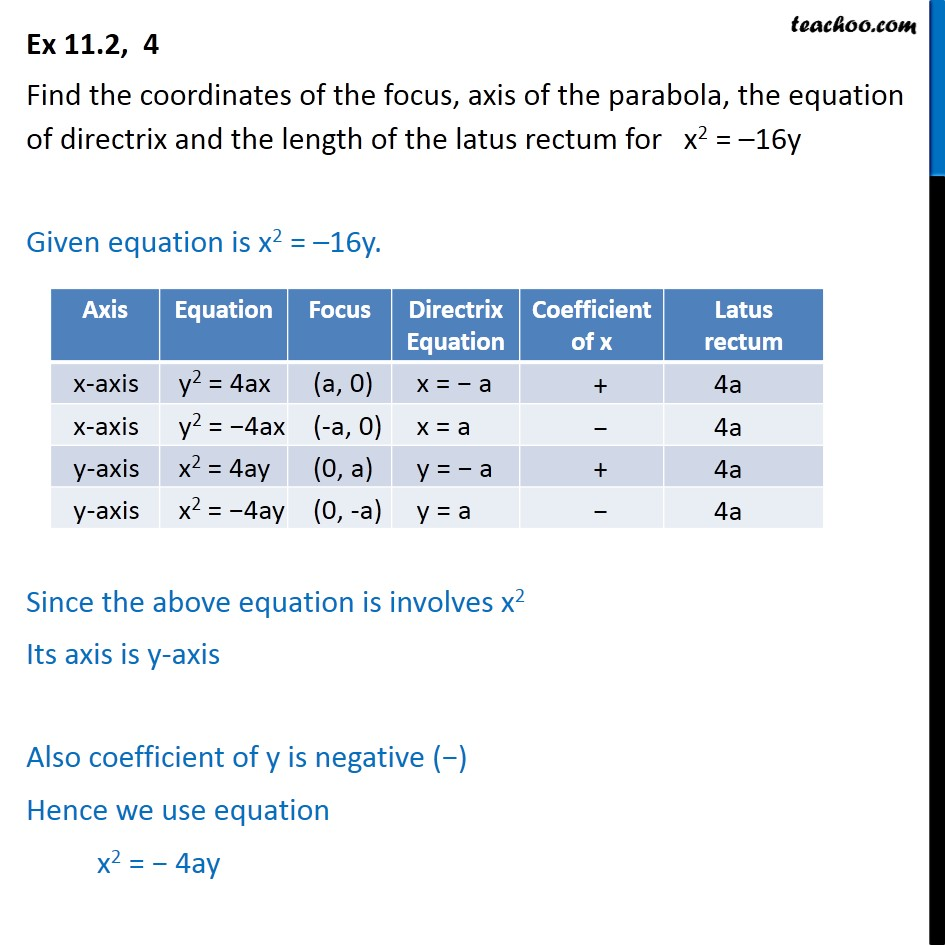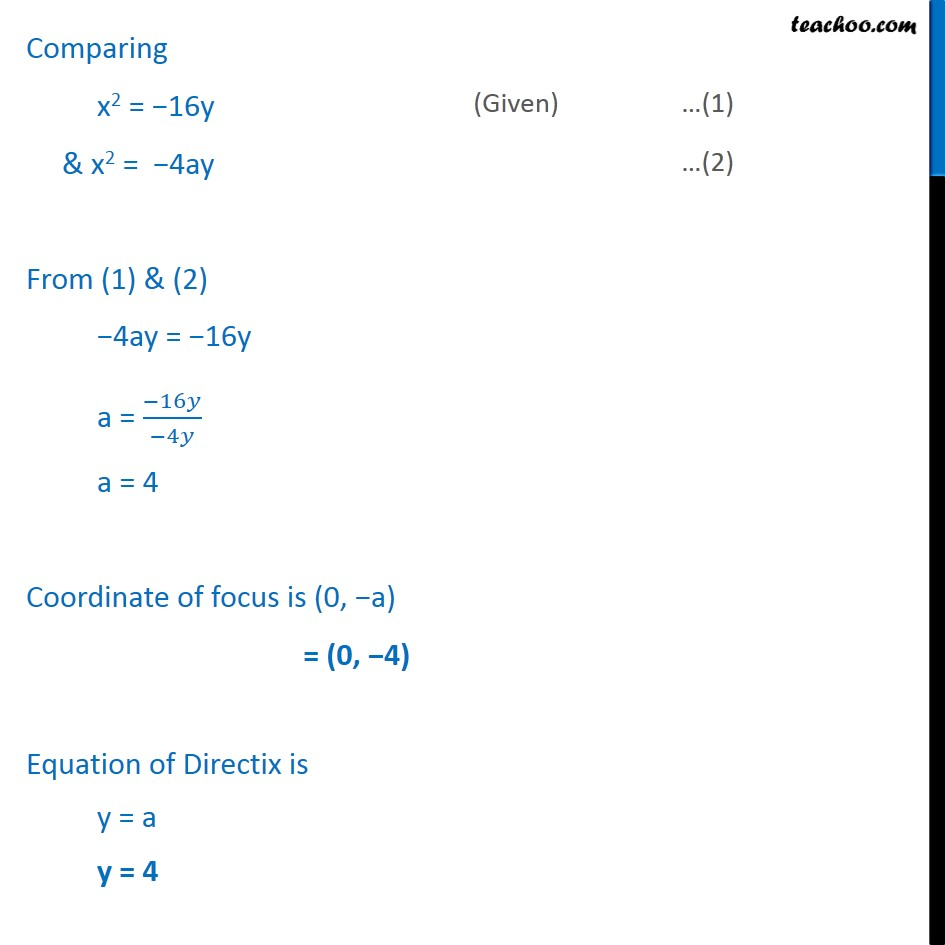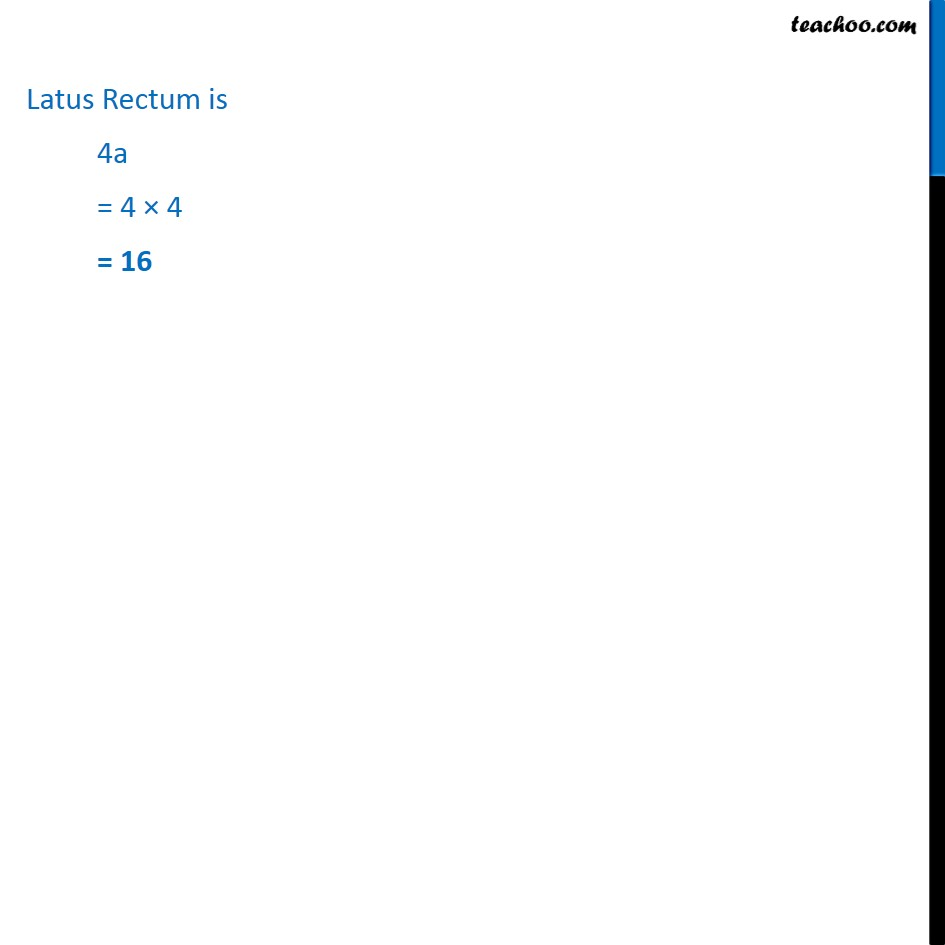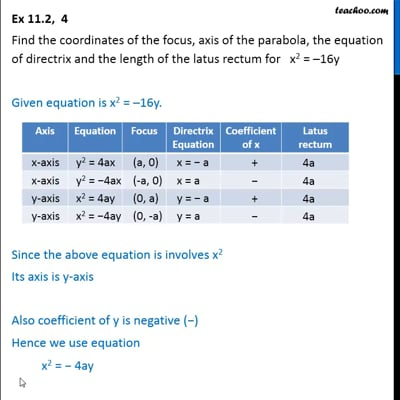Ex 11.2

Chapter 11 Class 11 Conic Sections
Serial order wiseThis video is only available for Teachoo black users

Solve all your doubts with Teachoo Black (new monthly pack available now!)

### Transcript

Ex 11.2, 4 Find the coordinates of the focus, axis of the parabola, the equation of directrix and the length of the latus rectum for x2 = 16y Given equation is x2 = 16y. Since the above equation is involves x2 Its axis is y-axis Also coefficient of y is negative ( ) Hence we use equation x2 = 4ay Latus Rectum is 4a = 4 4 = 16